# thesIt

Get the Flash Player to see this player.

#### Arif 11:33 am on January 13, 2010 | 0 | Thesis Road Map 1.3 | Tags: mind map, road map

•#### Arif 9:34 pm on August 24, 2010 | 0 | # | Tags: Geman 1992, standard citation

Geman S, Bienenstock E, Doursat R. 1992. Neural networks and the bias/variance dilemma. Neural Comput 4:1-58.

• #### Arif 3:07 pm on August 24, 2010 | 0 | # | Tags: error, Geman 1992, MSE, neural network, poor data, Silvert 1998, variance

Bias-variance dilemma (Geman et al., 1992). It can be demonstrated that the mean square value of the estimation error between the function to be modelled and the neural network consists of the sum of the (squared) bias and variance. With a neural network using a training set of fixed size, a small bias can only be achieved with a large variance (Haykin, 1994). This dilemma can be circumvented if the training set is made very large, but if the total amount of data is limited, this may not be possible.

• #### Arif 12:02 pm on August 24, 2010 | 0 | # | Tags: Rajkumar 2003, standard citation

Rajkumar T, Bardina J. 2003. Training data requirement for a neural network to predict aerodynamic coefficients. Di dalam: Bell AJ, Wickerhauser MV, Szu HH. Proceedings of Independent Component Analyses, Wavelets, and Neural Networks; Orlando: 22 Apr 2003. Orlando: SPIE. hlm (5102):92-103.

• #### Arif 5:41 am on August 24, 2010 | 0 | # | Tags: neural network, poor data, reference, Silvert 1998

Perhaps the greatest problem that is faced in most attempts to use artificial neural networks for ecological applications is that the quantity of data is often very limited. Although there are a few cases where large amounts of data are available, as in the case of remote sensing or observations based on automatic telemetry, it is far more common to have to deal with limited and irregularly spaced data, and the data may not always be strictly comparable due to variations in environmental conditions between sampling periods. In most situation the collection of field data is both time-consuming and expensive.

Since the training and testing of neural networks is very data-intensive, this poses serious obstacles to the development of neural network applications in ecology.

• #### Arif 5:36 am on August 24, 2010 | 0 | # | Tags: Silvert 1998, standard citation

Silvert W, Baptist M. 1998. Can neuronal networks be used in data-poor situations? Di dalam: Lek S, Guégan JF. Artificial Neuronal Networks: Application to Ecology and Evolution. Berlin: Springer-Verlag. hlm 241-248.

• #### Arif 1:03 pm on August 22, 2010 | 0 | # | Tags: Chan 1996, Levenberg-Marquardt, reference

Chan LW. 1996. Levenberg-Marquardt learning and regularization. Progress Neural Inform Processing 139-144.

iconip96.ps

• #### Arif 12:41 pm on August 22, 2010 | 0 | # | Tags: cross-validation, error, estimation, k-fold, Michaelsen 1987, reference, Stone 1974

Stone 1974 is referenced in:
Michaelsen J. 1987. Cross-validation in statistical climate forecast models. J Climate Applied Meteorology, 26:1589-1600

1520-0450(1987)026-1589-cviscf-2.0.co;2.pdf

Set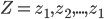$Z = z_{1}, z_{2},... , z_{1}$ consists of predictions and targets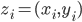$z_{i}=(x_{i}, y_{i})$

A set of prediction rule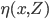$\eta (x,Z)$ will be used to predict y0 from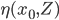$\eta (x_{0},Z)$

Let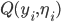$Q(y_{i},\eta_{i})$ be the accuracy.
by least squares this will usually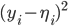$(y_{i}-\eta_{i})^{2}$
in other words expected Err is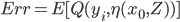$Err= E[Q(y_{i},\eta(x_{0},Z))]$

## MSE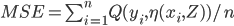$MSE= \sum_{i=1}^{n}Q(y_{i},\eta(x_{i},Z))/n$

In cross validation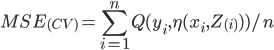$MSE_{(CV)}= \sum_{i=1}^{n}Q(y_{i},\eta(x_{i},Z_{(i)}))/n$

• #### Arif 12:19 pm on August 22, 2010 | 0 | # | Tags: basic, error, residual error, statistical error, wikipedia

If we assume a normally distributed population with mean μ and standard deviation σ, and take sample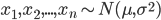$x_{1}, x_{2},..., x_{n}\sim N(\mu,\sigma^{2})$

statistical error is then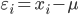$\varepsilon_{i}=x_{i}-\mu$

## Residual

while residual is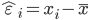$\hat{\varepsilon}_{i}=x_{i}-\bar{x}$

hat over the letter ε indicates an observable estimate of an unobservable quantity called ε.

• #### Arif 7:29 am on August 22, 2010 | 0 | # | Tags: basic, boxplot, outlier

Drawing boxplot from the following data, whiskers are determined from 1.5 IQR

 no datum drawn as 1 0.026 outlier 2 0.048 whisker low 3 0.070 Q1 4 0.072 5 0.076 Q2 6 0.084 7 0.086 8 0.099 Q3 9 0.102 10 0.103 whisker high

## Outlier

0.026 becomes outlier because 1.5IQR boundary (IQR = Q3 – Q1 = 0.02908) for the lower boundary is Q1 -1.5IQR = 0.0404, hence whisker is 0.048 which is more close to the box inside making 0.026 outlier.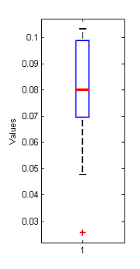boxplot-basic-concept-example.png

• #### Arif 6:43 am on August 22, 2010 | 0 | # | Tags: basic, viscosity, wikipedia

Viscosity is a measure of the resistance of a fluid which is being deformed by either shear stress or tensile stress.

The cgs physical unit for dynamic viscosity is the poise (P), more commonly expressed, particularly in ASTM standards, as centipoise (cP)